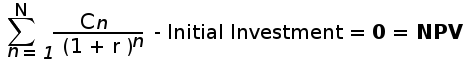# IRR Calculator

Calculate IRR (internal rate of return) online

 Initial Investment Cash Flow (negative or positive) Period 1 Period 2 Period 3 Period 4 Period 5

## What is IRRIRR stands for internal rate of return.The IRR is the interest rate (also known as the discount rate) that makes the NPV (Net Present Value) of all cash flows (both positive and negative) from a project or investment equal to zero. For instance, the investment's IRR is 25%, which is the rate that makes the net present value of the investment's cash flows equal to zero. It's an important financial metric often applied to analyse the desirability of a potential investment or a project. The higher IRR of an undertaken project indicates more worth pursuing and expected to give good return in future. IRR is sometimes also called as ERR or economic rate of return.

## IRR Calculation Formula

IRR or internal rate of return is calculated in terms of NPV or net present value. So, the formula for calculating IRR is same as NPV. Where NPV value is equal to zero.Where in the above formula :

N = total number of periods

n = positive integer

C = cash flow

r = internal rate of return

NPV = net present value

## How to Calculate IRR with example

Suppose a company plans to invest in a project with initial investment amount of \$10000. The expected net cash flow for three years are to be \$4500,\$4000 and \$5500 repectively. Let's calculate the internal rate of return for these period.

So the formula would be :

0 = C1/(1+r) + C2/(1+r)2 + C3/(1+r)3 - initial investment

After putting value in above equation, here's how the IRR equation looks:

0 = 4500/(1+r) + 4000/(1+r)2 + 5500/(1+r)3 - 10000

In this case, the r is 0.182, which makes IRR as 18.2%

Let's suppose the company is getting 10% return in other investment, then in the financial standpoint, the company must pursue this project which is likely to yield higher return of 18.2%.

Read more: Calculation of IRR in Excel

## IRR decision criterion

The general IRR decision rule is, if IRR of a project is greater than the company's minimum acceptable rate of return then the project should be taken. But if the IRR falls below the minimum acceptable rate of return then the project should be dropped. When comparing different projects, IRR can help us determine the project's likelihood of profit in future. A project with higher IRR should be given more weightage than project with lower IRR.

## IRR uses

IRR is used to evaluate desirability of a project or investment. Assuming all project require same up-front investment then the one with higher IRR is considered as desirable.

IRR plays good role in stock buyback programs inside big corporations. It shows if the investment in company's own stock is better than any other outside investment or use of the capital.

## Limitations of IRR

IRR should not be used to decide the mutually exclusive projects but to decide if a single project is worth pursuing.

Other limitation of IRR is that all cash flows are assumed to be re-invested at same rate but in real world this may change over long term.

IRR shouldn't be used to compare projects of different durations. A project of shorter duration may have higher IRR than the project of longer duration.